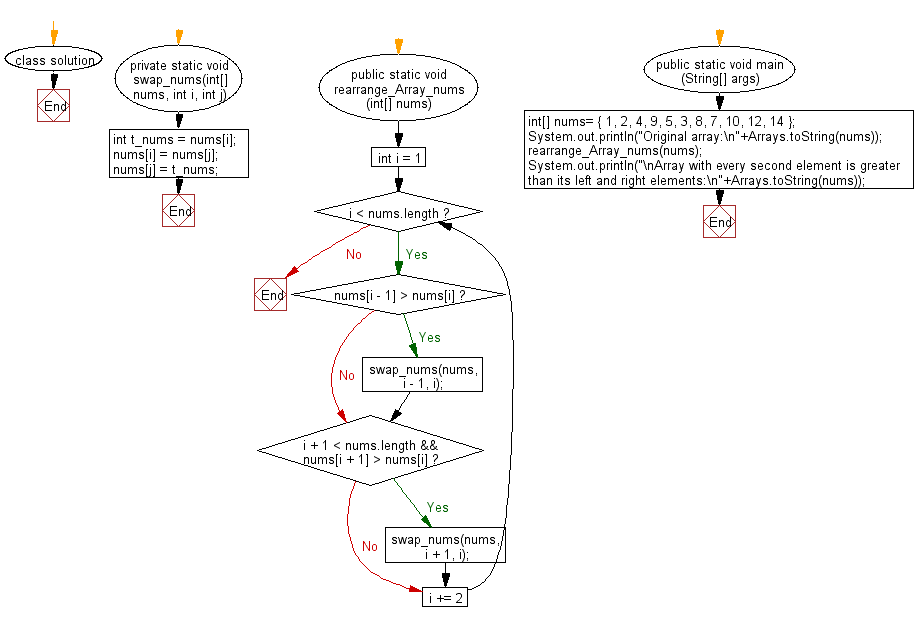﻿ Java: An array has every second element over the left, right# Java Array Exercises: Rearrange a given array of unique elements such that every second element of the array is greater than its left and right elements

## Java Array: Exercise-61 with Solution

Write a Java program to rearrange a given array of unique elements such that every second element of the array is greater than its left and right elements.

Example:
Input :
nums= { 1, 2, 4, 9, 5, 3, 8, 7, 10, 12, 14 }
Output:
Array with every second element is greater than its left and right elements:
[1, 4, 2, 9, 3, 8, 5, 10, 7, 14, 12]

Sample Solution:

Java Code:

``````import java.util.Arrays;

class solution
{
private static void swap_nums(int[] nums, int i, int j) {
int t_nums = nums[i];
nums[i] = nums[j];
nums[j] = t_nums;
}

public static void rearrange_Array_nums(int[] nums)
{
for (int i = 1; i < nums.length; i += 2)
{
if (nums[i - 1] > nums[i]) {
swap_nums(nums, i - 1, i);
}

if (i + 1 < nums.length && nums[i + 1] > nums[i]) {
swap_nums(nums, i + 1, i);
}
}
}

public static void main (String[] args)
{
int[] nums= { 1, 2, 4, 9, 5, 3, 8, 7, 10, 12, 14 };
System.out.println("Original array:\n"+Arrays.toString(nums));
rearrange_Array_nums(nums);
System.out.println("\nArray with every second element is greater than its left and right elements:\n"+Arrays.toString(nums));
}
}
```
```

Sample Output:

```Original array:
[1, 2, 4, 9, 5, 3, 8, 7, 10, 12, 14]

Array with every second element is greater than its left and right elements:
[1, 4, 2, 9, 3, 8, 5, 10, 7, 14, 12]
```

Flowchart:Java Code Editor:

Improve this sample solution and post your code through Disqus

What is the difficulty level of this exercise?

Test your Programming skills with w3resource's quiz.

﻿

## Java: Tips of the Day

countOccurrences

Counts the occurrences of a value in an array.

Use Arrays.stream().filter().count() to count total number of values that equals the specified value.

```public static long countOccurrences(int[] numbers, int value) {
return Arrays.stream(numbers)
.filter(number -> number == value)
.count();
}
```

Ref: https://bit.ly/3kCAgLb